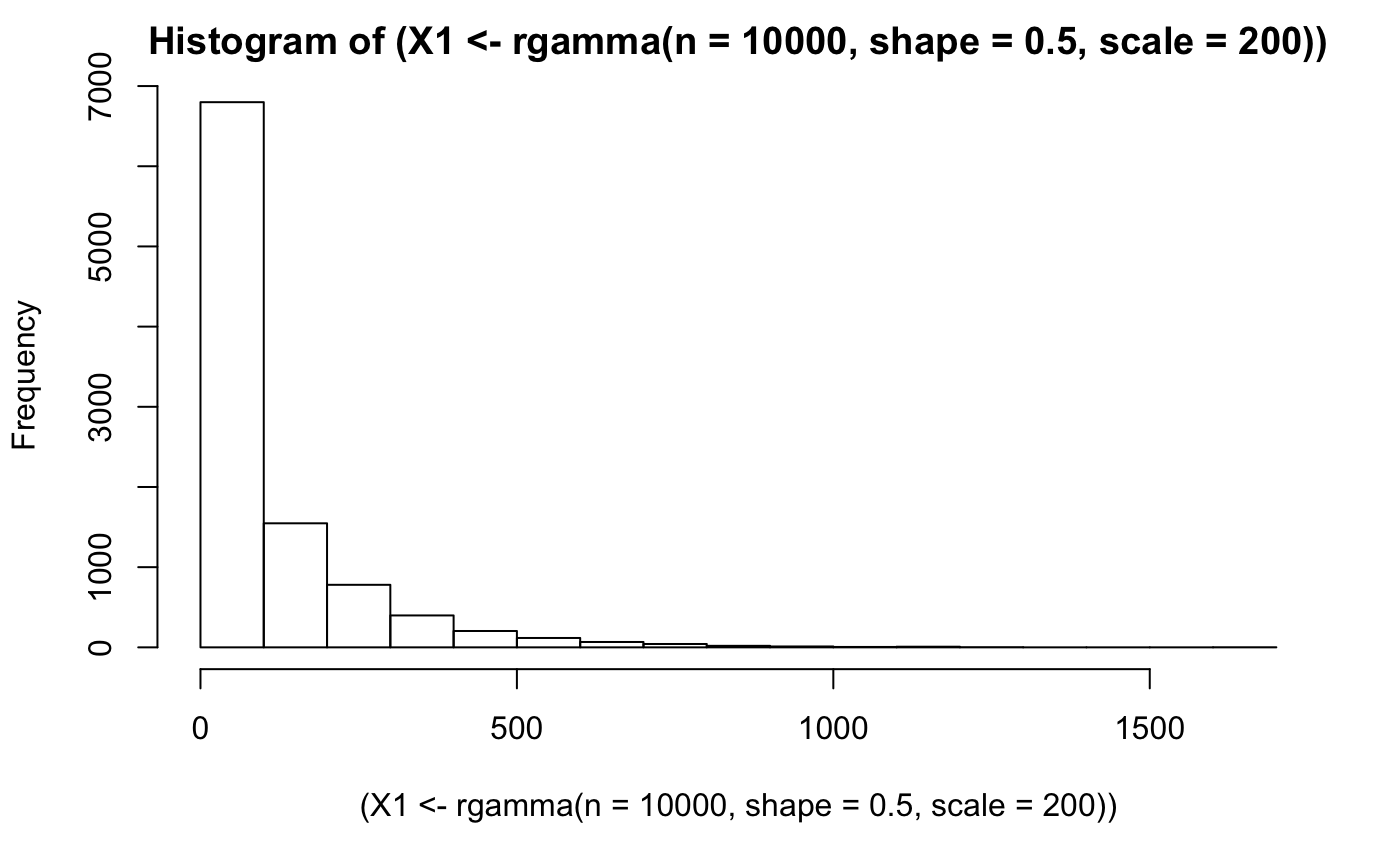Durations are commonly found in practice. For example the duration between visitors to a landing page, or api requests or signups, etc. In fact mixtures frequently occur together. The following shows how a mixture of two gamma distributions (often characterizing durations but sometimes other things) can be combined like `Gamma A / (Gamma A + Gamma B)` to form a beta distribution. The final graph shows the beta distribution fit to the two combined gamma distributions. The fit is perfect illustrating the relationships between two gammas and the beta distribution.

This is mind blowing and awesome to me. It makes sense durations and counts being different sides of the same coin and all, but this is a really cool and elegant mathematical relationship. #rstats https://t.co/kmsEhtIvKy pic.twitter.com/ctnxiOk0Fb

— Christopher Peters (@statwonk) November 12, 2017

This is a gamma distributed variable I’ll call “X1”,

``hist((X1 <- rgamma(n = 1e4, shape = 0.5, scale = 200)))``This is a gamma distributed variable I’ll call “X2”,

``hist((X2 <- rgamma(n = 1e4, shape = 3, scale = 200)))``